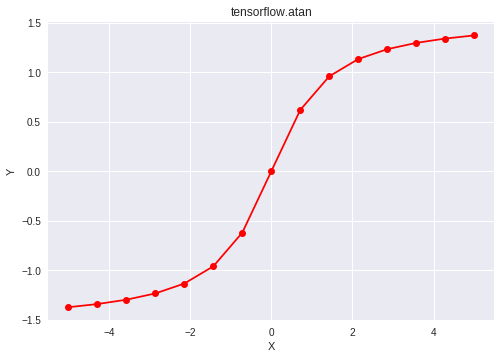Related Articles

# Python | Tensorflow atan() method

• Last Updated : 07 Dec, 2018

Tensorflow is an open-source machine learning library developed by Google. One of its applications is to develop deep neural networks.

The module `tensorflow.math` provides support for many basic mathematical operations. Function `tf.atan()` [alias `tf.math.atan`] provides support for the inverse tangent function in Tensorflow. It gives the output in radian form. The input type is tensor and if the input contains more than one element, element-wise inverse tangent is computed.

Syntax: tf.atan(x, name=None) or tf.math.atan(x, name=None)

Parameters:
x: A tensor of any of the following types: bfloat16, half, float32, float64, int32, int64, complex64, or complex128.
name (optional): The name for the operation.

Return type: A tensor with the same type as that of x.

Code #1:

 `# Importing the Tensorflow library``import` `tensorflow as tf`` ` `# A constant vector of size 6``a ``=` `tf.constant([``1.0``, ``-``0.5``, ``3.4``, ``0.2``, ``0.0``, ``-``2``],``                           ``dtype ``=` `tf.float32)`` ` `# Applying the atan function and``# storing the result in 'b'``b ``=` `tf.atan(a, name ``=``'atan'``)`` ` `# Initiating a Tensorflow session``with tf.Session() as sess:``    ``print``(``'Input type:'``, a)``    ``print``(``'Input:'``, sess.run(a))``    ``print``(``'Return type:'``, b)``    ``print``(``'Output:'``, sess.run(b))`

Output:

```Input type: Tensor("Const_8:0", shape=(6, ), dtype=float32)
Input: [ 1.  -0.5  3.4  0.2  0.  -2. ]
Return type: Tensor("atan:0", shape=(6, ), dtype=float32)
Output: [ 0.7853982  -0.4636476   1.2847449   0.19739556  0.         -1.1071488 ]
```

Code #2: Visualization

 `# Importing the Tensorflow library``import` `tensorflow as tf`` ` `# Importing the NumPy library``import` `numpy as np`` ` `# Importing the matplotlib.pylot function``import` `matplotlib.pyplot as plt`` ` `# A vector of size 15 with values from -5 to 5``a ``=` `np.linspace(``-``5``, ``5``, ``15``)`` ` `# Applying the inverse tangent function and``# storing the result in 'b'``b ``=` `tf.atan(a, name ``=``'atan'``)`` ` `# Initiating a Tensorflow session``with tf.Session() as sess:``    ``print``(``'Input:'``, a)``    ``print``(``'Output:'``, sess.run(b))``    ``plt.plot(a, sess.run(b), color ``=` `'red'``, marker ``=` `"o"``) ``    ``plt.title(``"tensorflow.atan"``) ``    ``plt.xlabel(``"X"``) ``    ``plt.ylabel(``"Y"``) `` ` `    ``plt.show()`

Output:

```Input: [-5.         -4.28571429 -3.57142857 -2.85714286 -2.14285714 -1.42857143
-0.71428571  0.          0.71428571  1.42857143  2.14285714  2.85714286
3.57142857  4.28571429  5.        ]
Output: [-1.37340077 -1.34156439 -1.29778762 -1.23412151 -1.13416917 -0.96007036
-0.62024949  0.          0.62024949  0.96007036  1.13416917  1.23412151
1.29778762  1.34156439  1.37340077]
```Attention geek! Strengthen your foundations with the Python Programming Foundation Course and learn the basics.

To begin with, your interview preparations Enhance your Data Structures concepts with the Python DS Course. And to begin with your Machine Learning Journey, join the Machine Learning – Basic Level Course

My Personal Notes arrow_drop_up